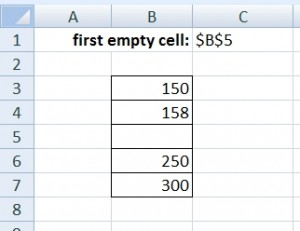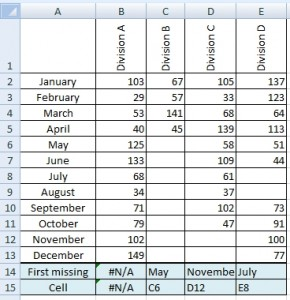# Address of the first empty cell in a column

In some cases it is useful to know the address of the first empty cell in a column. This is a way to do this without macros. Write the following formula and press CTRL+SHIFT+ENTER. Excel adds braces { } and saves it as array formula.

```=ADDRESS(ROW(INDEX(firstCell:lastCell,
MATCH(TRUE,LEN(firstCell:lastCell)=0,0),1)),column number)```

## Example 1### Solution

`{=ADDRESS(ROW(INDEX(B3:B7,MATCH(TRUE,LEN(B3:B7)=0,0),1)),2)}`

## Example 2When (in which month) the division interrupted filling data? We are searching for the first blank cell in the column of division A, B, C and D. Formula for the Division A:

`{=INDEX(\$A\$2:\$A\$13,MATCH(TRUE,LEN(B2:B13)=0,0),1)}`

Note: Value #N/A means that there is no missing data.

And which cell is it?

`{=ADDRESS(ROW(INDEX(B2:B13,MATCH(TRUE,LEN(B2:B13)=0,0),1)),COLUMN(B2),4)}`

1.2.